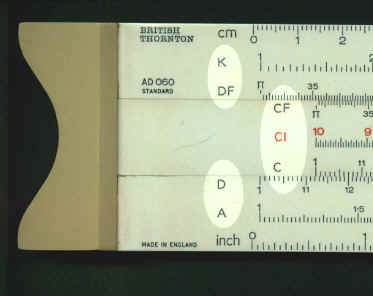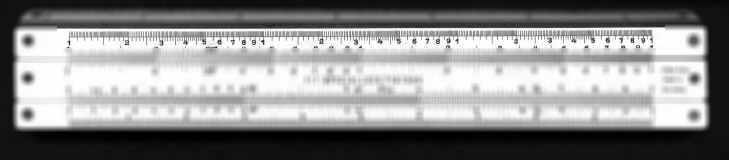Scales

A standard naming system has developed for scales over the years. For example most calculations are done using C and D scales, the A and B scales usually represent the values on the C and D scales squared, and so on. Not all makers, particularly earlier ones, respected this naming convention or even gave their scales any name at all. The following table shows the normal designation and alternative names commonly found. If you have a scale whose name is not on the list, look at the column "Numbering (for identification)" which should enable you to identify it.

You should be aware that since, for most of the scales, the position of the decimal point is not clear from the scale marking some scales may be marked as, for example, p to p. A scale like this would start at 3.14, have numbers increasing to 9, a 1 in the middle of the scale and then numbers increasing to 3.14.

The numbering is given from left to right so, for example, reciprocal scales are shown as running from 10 to 1.

This image shows a rule which follows the standard naming convention.The next image shows a rule on which the scales are not named but, for example, the upper scale of the stock can be identified as a cube (K) scale as it has 1 to 1 repeated 3 times. (The cursor has been removed for clarity).Normal Designation Alternative Designation Numbering (for identification) Uses Notes A x2 1 to 100, 1 to 10 repeated twice Squares and square roots (on stock) B x2 1 to 100, 1 to 10 repeated twice Squares and square roots (on slide) C x 1 to 10 Multiplication and division (on slide) C1,C2 Ö10 to Ö10 C scales shifted by Ö10 CI RC, 1/x, R 10 to 1 Reciprocals (on slide) CF px Pi to Pi Multiplication and division by pi CIF Pi to Pi Multiplication and division by pi D x 1 to 10 Multiplication and division (on stock) D1,D2 Ö10 to Ö10 D scales shifted by Ö10 DF px Pi to Pi Multiplication and division by pi DI 10 to 1 Reciprocals (on stock) Dynamo/Motor 20 to 100 to 20 Electrical efficiency Often in well of stock Fahr/Cent Various Celcius (centigrade) to Fahrenheit conversion, Change of resistance with temperature. Often on electrical rules. ISd 1.0 to 0.0 (short scale on slide) Inverse sines On PIC/Thornton rules ITd 0 to 1.76 (short scale on slide) Inverse tangents On PIC/Thornton rules K x3, d 3 1 to 1000, 1 to 1 repeated 3 times Cubes and cube roots L lg x,log 0.0 to 1.0 0.0 to 0.5 and 0.5 to 1 Logarithms (base 10) Two ranges are only for use with W scales. LC 25000 to 2.5 (2px)2 For electronic calculations (Cooke). LG H 2.5 to 2.5 1/(2px)2 For electronic calculations (Pickett). Ln 0 to 2.3 Logarithms (base e) LL00 e-x .999 to .99 Raising to a power (x<1) LL01 e-0.1x .99 to .91 Raising to a power (x<1) LL02 e-0.01x .91 to .37 Raising to a power (x<1) LL03 e-0.001x .37 to .0001 Raising to a power (x<1) LL0 e0.001x 1.001 to 1.01 Raising to a power (x>1) LL1 e0.01x 1.01 to 1.12 Raising to a power (x>1) LL2 E1,e0.1x ,LU 1.1 to 3.0 (slight variations in upper and lower bound) Raising to a power (x>1) LL3 E2,ex ,LL 2.6 to 105 (slight variations in upper and lower bound) Raising to a power (x>1) P f(x),Ö(1-s2),Ö(1-x2) .995 to 0 Right angled triangles S sin 5.5° to 90° Sine Sd sin 0° to 90° (short scale on slide) Sines On PIC/Thornton rules Sh SH, Sh1, Sh2 0.1 to 0.89 and 0.89 to 3.0 Hyperbolic sine Sq1,Sq2 Ö 1 to 3.16 and 3.16 to 10 Square and square roots Sq on K&E and Ö on Pickett. ST S&T, sin tan, arc, SRT, s-t .55° to 6°, 40' to 5° 45' (slight variations in upper and lower bound) Sin and tangent for small angles T tan, T1, T2,tg 5.5° to 45° sometimes also 45° to 84.3° Tangent Td tan 0° to 60° (short scale on slide) Tangents On PIC/Thornton rules Th TH 0.1 to 3.0 Hyperbolic tan Volt 05. to 10 Voltage loss Often in well of stock W1,W1',W2,W2' P1,P2,Q1,Q2 Ö10x and Öx 2.8 to 11 and 0.9 to 3.5 or1 to 3.16 and 3.16 to 10 20" scales on slider of 10" rule or 10" scales on 5" rule Extended range on Faber Castell 2/83N Y 10% to 0% (small scale on stock) Interest rate calculations On PIC/Thornton rules Z 10 to 0  (small scale on stock) Interest rate calculations On PIC/Thornton rules 2p fx 2p 1.6 to 1.6 1/(2px) For electronic calculations. square root 3Ö cube root 1 to 2.16, 2.16 to 4.64 and 4.64 to 10 Cube and cube roots Mainly on Pickett rules

An explanation of how to read the scales is given here.